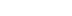/
article

# Union Find algorithm in practice

I would like to share possible ways of usage of Union Find in the city builder and solitaire game.

Problem 1
We have a random amount of cards on the table. We can only collect cards that differ less or more by one rank. For example, in Sequence 1 we can collect 2 -> 3 -> 4. And in Sequence 2: possible way is J -> 10 -> 9 -> 8 -> 9. And we would like to define how many sequences like that we can collect.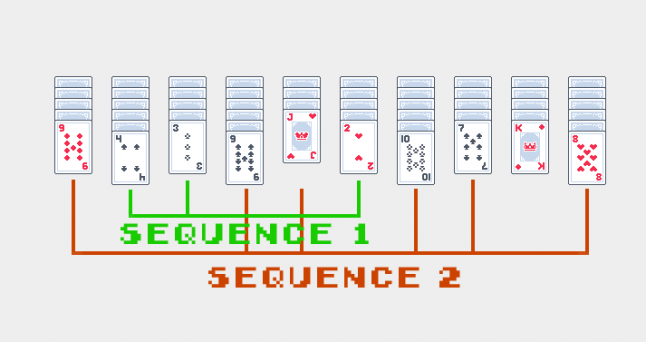Problem 2
Imagine that we have a terrain, this terrain consists of walkable and non-walkable objects. Non-walkable objects can divide our terrain into closed territories and we would like to perform a check can character walk from one part of the terrain to another.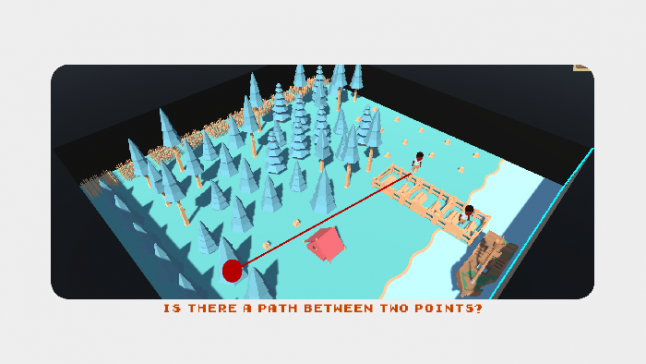At the first sight, these two problems have two different solutions. In one case you need to recursively check all cards, and in another, you need to perform pathfinding from point a to point b. But these approaches will be a naive solution. That will take an eternity on pretty large data sets.

So what I would like to use here is called Union Find or Disjoint-set data structure. There are plenty of implementations of this algorithm but I would like to use the simplest and non-optimized but still super fast (compared to naive solutions) version of the algorithm, also called Quick Find.

Operation 1: Union

Let’s implement the Union operation. To understand how it works we will go through a step by step process.

To implement union operation, the first thing that we need to do is to create an auxiliary array size of N. Where N is the number of items that you have. Each item should have an integer id that will correspond to the array index.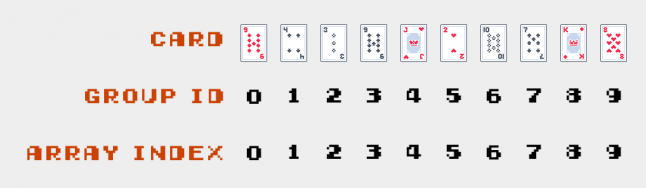``````private int[] _ids;

public QuickFind(int n)
{
_ids = new int[n];

for (var i = 0; i < n; i++)
{
_ids[i] = i;
}
}``````

In our auxiliary array, the card id will be basically a group id.

So, to unite two cards for example 4 and 3. They should share the same group. So if we will treat card 4 as a parent, that means that the group id of card 3 will be one.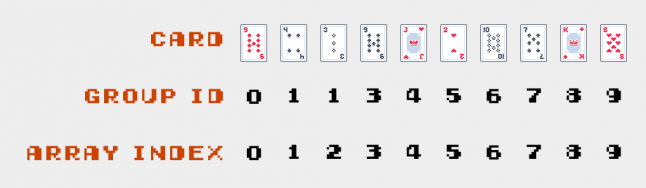Next, let’s unite cards 9 and 10. We will treat card 9 as a parent and so card 10 will have the id 0.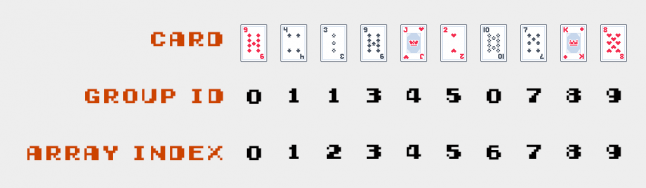As you already noticed we started from pretty random cards, its because there is no reason to follow any order.

Let’s unite cards 7 and 8! We will treat card 7 as a parent, so card 8 will now have group 7.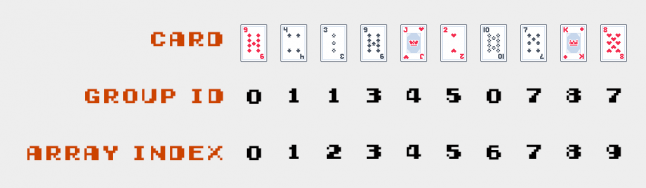For the next step, I would like to unite cards 9, 10, 7, and 8. To make this operation we can unite cards 9 and 7 or 10 and 8 or 9 and 8 or 10 and 8. It’s doesn’t really matter as long as the group shares the same id. So, we will take a parent of group 9, 10 which is 0, and set it for cards 7 and 9 via a loop.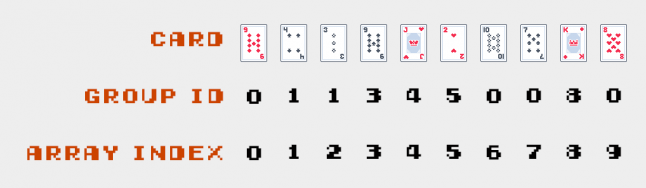We just look at the basic cases of the Union operation. Now let’s look at the code.

``````public void Union(int p, int q)
{
var pId = _ids[p];
var qId = _ids[q];

for (var i = 0; i < _ids.Length; i++)
{
if (_ids[i] == pId)
{
_ids[i] = qId;
}
}
}``````

The time complexity of union operation is O(N).

Operation 2: Find
The find operation is simple as heck. We just take value by index and check if they equal, if so, then the group is connected.

``````public bool Connected(int p, int q)
{
return _ids[p] == _ids[q];
}``````

Time complexity O(1).

Union find in a solitaire game
Let’s look again at the image that we saw at the beginning of the article.Basically, we have three sequences here. Because the King is a group itself. So, how exactly are we going to figure out those groups?

We need to have two loops. We are going to iterate through all cards and then we will try to unite them with the other cards. If cards are already united: do nothing.

After we united all possible cards. We can go through the cards again and calculate the number of groups, calculate the number of cards inside each group, take the bigger or smaller group. You name it.

``````var quickFind = new QuickFind(cardsOnTheTable.Length);

foreach (var cardA in cardsOnTheTable)
{
foreach (var cardB in cardsOnTheTable)
{
if (quickFind.Connected(cardA.Id, cardB.Id))
{
continue;
}

if (Math.Abs(cardA.Rank - cardB.Rank) == 1)
{
quickFind.Union(cardA.Id, cardB.Id);
}
}
}

var groups = new Dictionary<int, int>();

foreach (var card in cardsOnTheTable)
{
var groupId = quickFind.Find(card.Id);
groups[groupId] = groups.Contains(groupId) ? groups[groupId] + 1 : 1;
}``````

Union find to check if there is a path
To make this operation, let’s rotate our game field, so it will look like a matrix.

 T T T T C G T T T T T

The T symbol here stands for an impassable tree, the C symbol for the character, and G is for goal. As with the card example, let’s set for each cell an Id.

 0T 1T 2T 3 4 5 6 7T 8C 9 10G 11 12T 13 14 15 16 17T 18 19 20 21 22T 23T 24T

Then we are going to iterate through each cell. Each time we will try to unite the current cell with adjacent cells. But only if the cell itself and adjacent cell are not walkable.

 0T 1T 2T 3 4 5 6 7T 8C 9 10G 11 12T 13 14 15 16 17T 18 19 20 21 22T 23T 24T

Let’s skip 3 cells since they are not walkable and move to cell 3. Let’s unite adjacent cells. The adjacent cells will be left, right, top and bottom. In this case, we will unite cells 3 and 4.

 0T 1T 2T 3 3 5 6 7T 8C 9 10G 11 12T 13 14 15 16 17T 18 19 20 21 22T 23T 24T

Next, let’s move to cell 5. We will unite 5, 10, and 6.

 0T 1T 2T 3 3 5 5 7T 8C 9 5G 11 12T 13 14 15 16 17T 18 19 20 21 22T 23T 24T

Next, we will unite cells 8, 3, 13, and 9.

 0T 1T 2T 8 8 5 5 7T 8C 8 5G 11 12T 8 14 15 16 17T 18 19 20 21 22T 23T 24T

And let’s fill all cells.

 0T 1T 2T 19 19 21 21 7T 19C 19 21G 21 12T 19 19 21 21 17T 19 19 21 21 22T 23T 24T

As you can see, cells have two groups now. So we can easily check if there is a path between two cells.

And here is a pseudocode for this problem.

``````var quickFind = new QuickFind(amountOfRows * amountOfCols);

for (var row = 0; row < amountOfRows; row++)
{
for (var col = 0; col < amountOfCols; col++)
{
if (matrix[row, col].IsWalkable)
{
}
}
}

void UniteCellWithAdjacent(Cell[,] matrix, int row, int col, QuickFind quickFind)
{
if (matrix[row - 1, col].IsWalkable) quickFind.Unite(matrix[row, col], matrix[row - 1, col]);
if (matrix[row + 1, col].IsWalkable) quickFind.Unite(matrix[row, col], matrix[row + 1, col]);
if (matrix[row, col - 1].IsWalkable) quickFind.Unite(matrix[row, col], matrix[row, col - 1]);
if (matrix[row, col + 1].IsWalkable) quickFind.Unite(matrix[row, col], matrix[row, col + 1]);
}``````

You can do a lot of improvements here: move code that unites two cells to separate method and check if cells are walkable or already united, you can change the logic of adjacent cells for hexagon tiles, or if your character can move diagonal, then support diagonal cells, etc.

Conclusion

As you can see the Union Find or Disjoint Set data structure is a powerful thing that can help solve complex problems in a reasonable time. We didn’t cover any optimizations of Union Find and took the simplest algorithm possible. The optimized version you can find on my GitHub: https://github.com/thenitro/algorithms/tree/master/Structures/UnionFind. Feel free to dive deeper into the topic. Algorithms are fun.

### Latest Jobs

#### Treyarch

Playa Vista, California
6.20.22
Audio Engineer

#### Digital Extremes

6.20.22
Communications Director

6.20.22
Senior Producer

#### Build a Rocket Boy Games

Edinburgh, Scotland
6.20.22
More Jobs